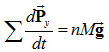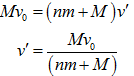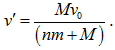×#### Thank you for registering.

One of our academic counsellors will contact you within 1 working day.

Click to Chat

1800-1023-196

+91-120-4616500

CART 0

• 0

MY CART (5)

Use Coupon: CART20 and get 20% off on all online Study Material

ITEM
DETAILS
MRP
DISCOUNT
FINAL PRICE
Total Price: Rs.

There are no items in this cart.
Continue Shopping
```
An isolated rail car of mass M is moving along a straight, frictionless track at an initial speed Vo. The car is passing under a bridge when a crate filled with N bowling balls, each of mass m, is dropped from the bridge into the bed of the rail car. The crate splits open and the bowling balls bounce around inside the rail car, but none of them fall out. (a) Is the momentum of the rail car + bowling balls system conserved in this collision? (A) Yes, the momentum is completely conserved. (B) Only the momentum component in the vertical direction is conserved. (C) Only the momentum component parallel to the track is conserved. (D) No components are conserved. (b) What is the average speed of the rail car + bowling balls system sometime after the collision? (A) (M + Nm)v 0 /M (B) MV o /(Nm + M) (C) Nmv o /M (D) The speed cannot be determined because there is not enough information.
An isolated rail car of mass M is moving along a straight, frictionless track at an initial speed Vo. The car is passing under a bridge when a crate filled with N bowling balls, each of mass m, is dropped from the bridge into the bed of the rail car. The crate splits open and the bowling balls bounce around inside the rail car, but none of them fall out.(a) Is the momentum of the rail car + bowling balls system conserved in this collision?                (A) Yes, the momentum is completely conserved.(B) Only the momentum component in the vertical direction is conserved.(C) Only the momentum component parallel to the track is conserved.(D) No components are conserved.(b) What is the average speed of the rail car + bowling balls system sometime after the collision?(A) (M + Nm)v0/M(B) MVo/(Nm + M)(C) Nmvo/M(D) The speed cannot be determined because there is not enough information.

```
5 years ago396 Points
```							(a)(C) only the momentum component parallel to track is conserved.(b)(D) the speed cannot be determined because there is not enough information.(a)When the ball is up in the air, the net external force acting on the balls is the gravitational force, and therefore the rate of change of momentum for a ball with mass M can be written as:The bed of the railcar, initially at rest, remain in the same state when the balls are up in the air, and therefore the rate of change of total momentum in the vertical direction for the entire system is equal to the rate of change of momentum (in the vertical direction) for all the balls, that is,When the balls collide with the bed of the railcar, the magnitude of internal forces is larger than the external gravitational force, and being the action-reaction pair, the effect of internal forces cancel out, and the rate of change of momentum for the balls in collision is zero.This breaks down the conservation of momentum in the vertical direction.However, no force acts on the railcar and the balls in the horizontal direction, therefore the rate of change of momentum of the system remains zero at all times.Therefore, (C) is the correct option, and the rest are ruled out.(b)Assume that the final speed of the rail car + bowling balls in the horizontal direction is v’ , then from conservation of linear momentum, one can writeHowever, the balls also move in the vertical direction, where momentum is not conserved, and their final velocity depends on the value of time t, after which the velocity of the system is considered.Therefore, without any assumptions, one cannot calculate the average speed of the railcar + bowling balls system, but if one assumes the vertical component of momentum of the bouncing balls (some time after collision) to be negligible as compared to the horizontal momentum of the system, the average speed of the system can be equated toWe choose the answer without making any assumption. Thus (D) is the correct option, and the rest are ruled out.
```
5 years ago
Think You Can Provide A Better Answer ?

## Other Related Questions on Mechanics

View all Questions »### Course Features

• 101 Video Lectures
• Revision Notes
• Previous Year Papers
• Mind Map
• Study Planner
• NCERT Solutions
• Discussion Forum
• Test paper with Video Solution### Course Features

• 110 Video Lectures
• Revision Notes
• Test paper with Video Solution
• Mind Map
• Study Planner
• NCERT Solutions
• Discussion Forum
• Previous Year Exam Questions